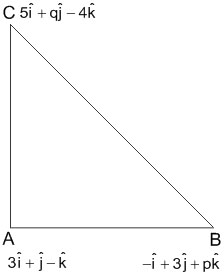#### In a triangle ABC, right angled at the vertex A, if the position vectors of A, B and C are respectivelythen the point (p, q) lies on a line : Option 1) parallel to x-axis. Option 2) parallel to y-axis. Option 3) making an acute angle with the positive direction of x-axis. Option 4) making an obtuse angle with the positive direction of x-axis

As we learnt in

Scalar Product of two vectors -- whereinis the angle between the vectorsReplace (p,q) with (x,y)

3x-2y+13=0Acute angle with +x-axis

Option 1)

parallel to x-axis.

This option is incorrect.

Option 2)

parallel to y-axis.

This option is incorrect.

Option 3)

making an acute angle with the positive direction of x-axis.

This option is correct.

Option 4)

making an obtuse angle with the positive direction of x-axis

This option is incorrect.

#### Sabhrant Ambastha